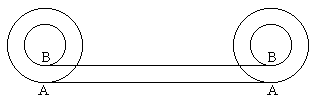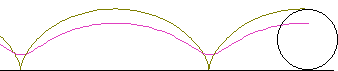# Train wheel quandaryConsider two wheels of different radii on the same axis rigidly attached to each other so that when one completes a full revolution so does another. Now let's imagine that a bigger wheel rolls along a straight line (see the cycloids page.) After one complete revolution the bottom most point A of the big circle will traverse the distance AA equal to the circumference of the big circle. But then the lowest point B of the small circle will also make one complete revolution and thus traverse the distance BB equal to the circumference of the small circle. But, from the diagram, AA = BB which is of course absurd.The fallacy in the previous argument becomes obvious on observing how the cycloids are actually formed (drawn). It's as wrong to claim that the point B traversed the distance BB as is to claim that the point A traversed the distance AA. Furthermore, strange as it may sound, the distances covered by the two points are not at all equal. A "travels farther" than B!

To better understand how this is possible, note that the wheel takes part in two distinct motions (compare this to the Two coins puzzle.) It moves along a straight line (AA is actually the distance covered by the common center of the two circles) and it rotates around its own center. It can be shown (and is planned to be shown later on) that a combination of two such motions is a rotation around some other point. Generally, speaking, as a result of such a rotation, points on a geometric shape will cover different distances.

## References

1. J.R. Newman, The World of Mathematics, v3, Simon and Schuster, NY, 1956